# Kaggle Titanic（泰坦尼克号幸存预测）## 一、提出问题

Titanic是 Kaggle 上的一个竞赛。属于入门级比赛，在 Kaggle 提供的数据集中，共有1309名乘客数据，其中891是已知存活情况，剩下418则是需要进行分析预测的。

• gender_submission.csv：提交结果案例（提交结果）
• test.csv：测试数据
• train.csv：训练数据

## 二、理解数据

``````train_set = pd.read_csv('./datasets/titanic/train.csv')
test_set = pd.read_csv('./datasets/titanic/test.csv')
``````

``````train_set.head()
``````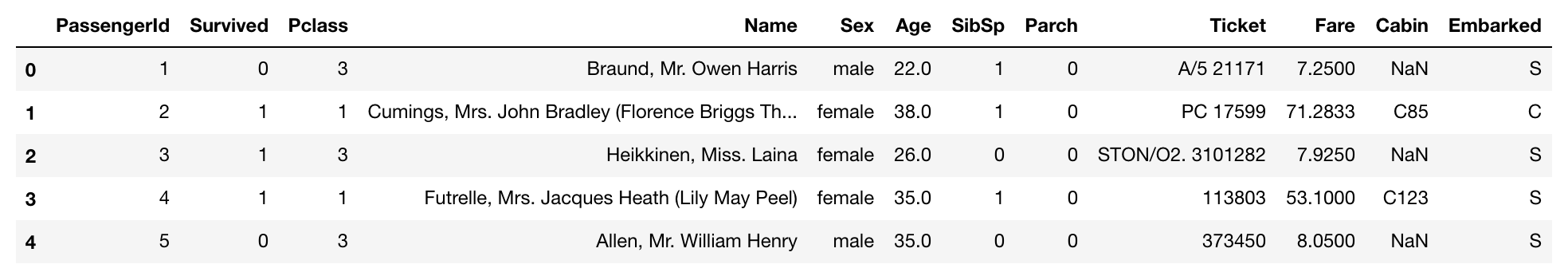• PassengerId：乘客的ID（对预测没有用处）
• survival：是否存活，0表示死亡，1表示存活
• pclass：船票的类别，可以用来表示乘客的经济情况，1 = 1st, 2 = 2nd, 3 = 3rd
• sex：乘客性别
• Age：乘客年龄
• sibsp：船上兄弟姐妹及配偶的个数
• parch：船上父母或子女的个数
• ticket：船票号
• fare：船票价格
• cabin：客舱号码
• embarked：登船口岸，C = Cherbourg, Q = Queenstown, S = Southampton

``````train_set.info()
``````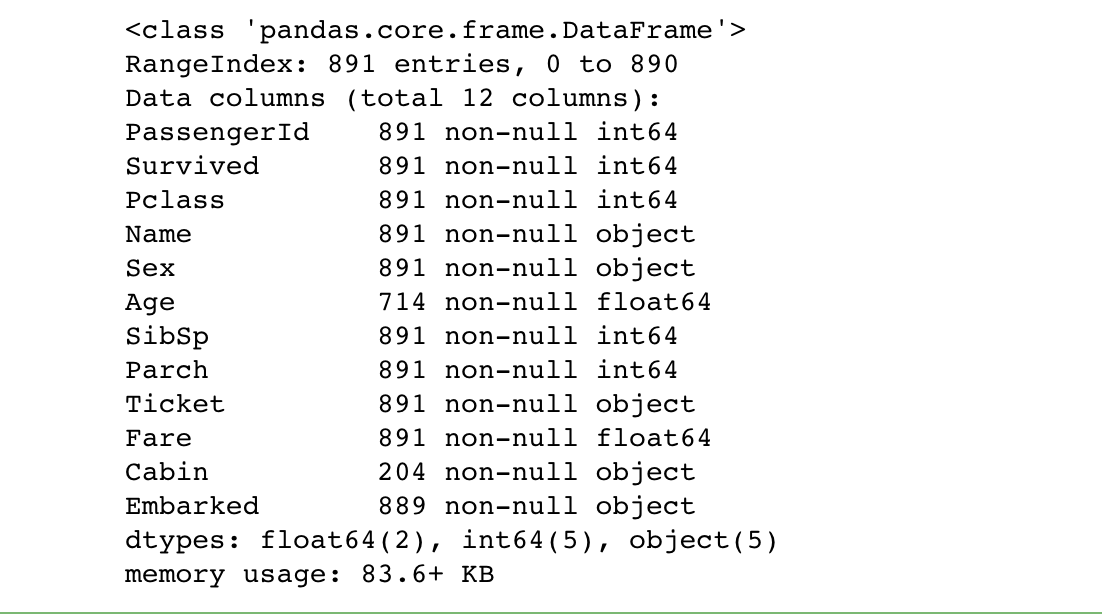``````train_set.describe()
``````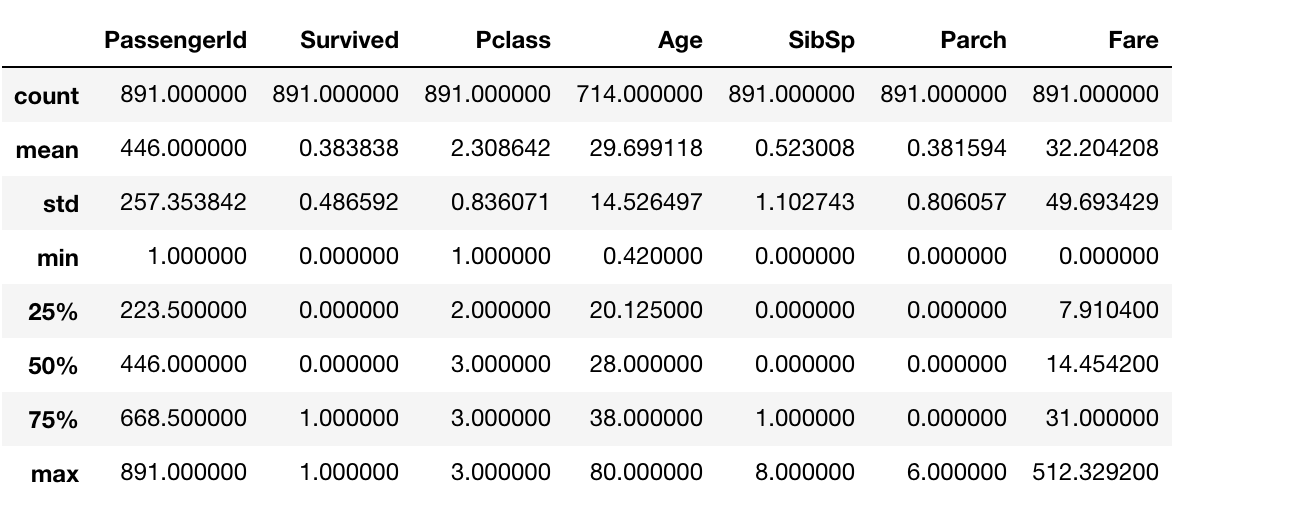## 三、数据清洗

### 2.特征工程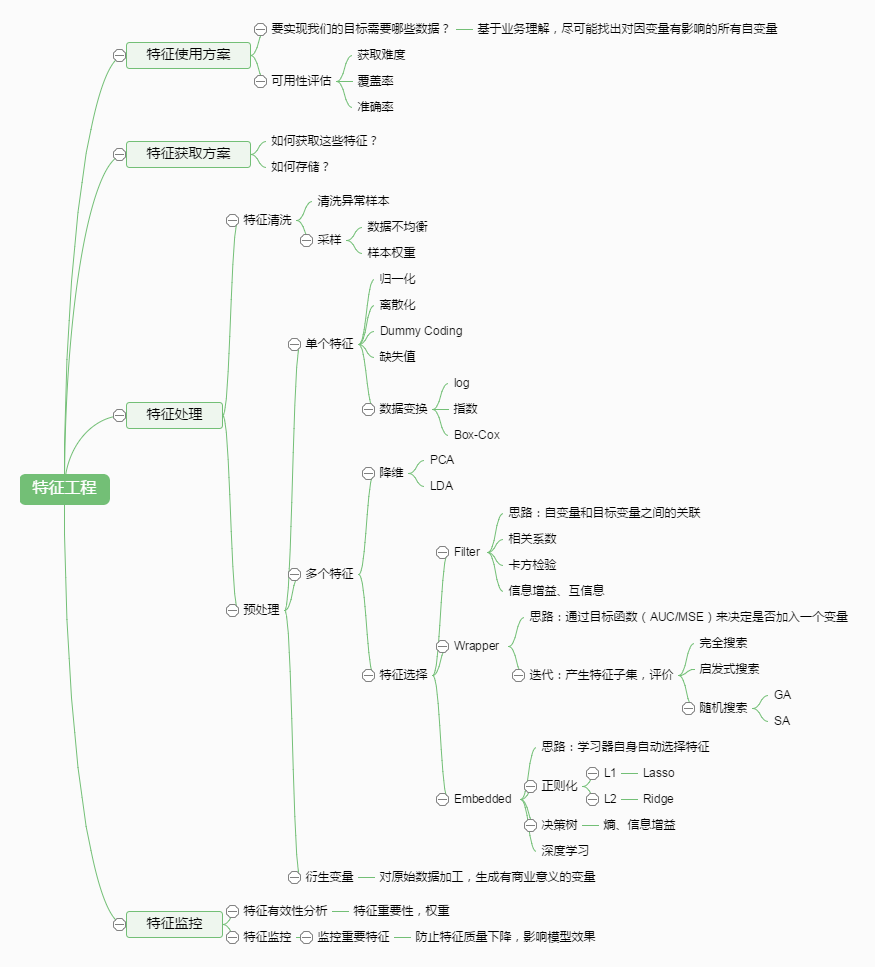``````from sklearn.base import BaseEstimator, TransformerMixin

# A class to select numerical or categorical columns
# since Scikit-Learn doesn't handle DataFrames yet
class DataFrameSelector(BaseEstimator, TransformerMixin):
def __init__(self, attribute_names):
self.attribute_names = attribute_names
def fit(self, X, y=None):
return self
def transform(self, X):
return X[self.attribute_names]
``````

``````from sklearn.pipeline import Pipeline
from sklearn.preprocessing import Imputer

# 按中位数进行填充
imputer = Imputer(strategy="median")

num_pipeline = Pipeline([
("select_numeric", DataFrameSelector(["Age", "SibSp", "Parch", "Fare"])),
("imputer", Imputer(strategy="median")),
])
``````

``````num_pipeline.fit_transform(train_set)
``````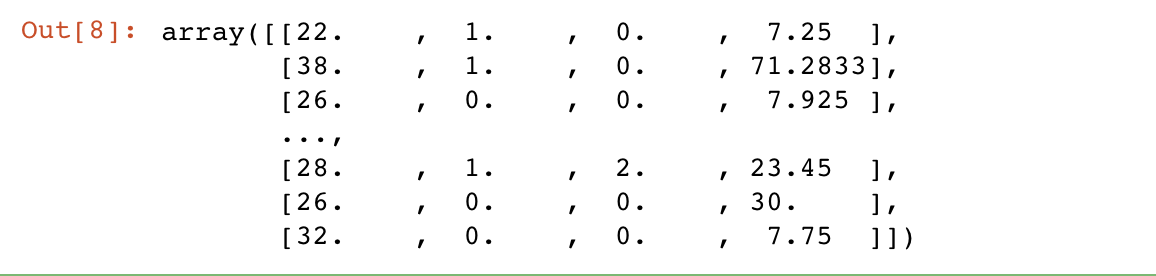``````class MostFrequentImputer(BaseEstimator, TransformerMixin):
def fit(self, X, y=None):
self.most_frequent_ = pd.Series([X[c].value_counts().index for c in X],
index=X.columns)
return self
def transform(self, X, y=None):
return X.fillna(self.most_frequent_)

from future_encoders import OneHotEncoder

cat_pipeline = Pipeline([
("select_cat", DataFrameSelector(["Pclass", "Sex", "Embarked"])),
("imputer", MostFrequentImputer()),
("cat_encoder", OneHotEncoder(sparse=False)),
])
``````

``````cat_pipeline.fit_transform(train_set)
``````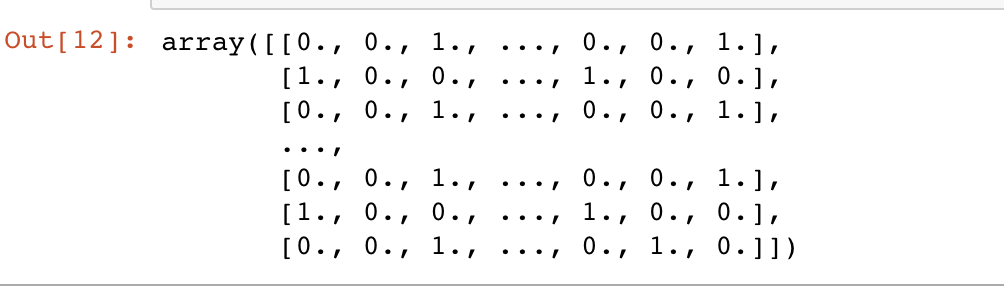## 四、构建模型

### 1.获取训练数据

``````# 将数据的清洗操作做为一个完整的管道操作来进行
from sklearn.pipeline import FeatureUnion
preprocess_pipeline = FeatureUnion(transformer_list=[
("num_pipeline", num_pipeline),
("cat_pipeline", cat_pipeline),
])
``````

``````X_train = preprocess_pipeline.fit_transform(train_set)
X_train
``````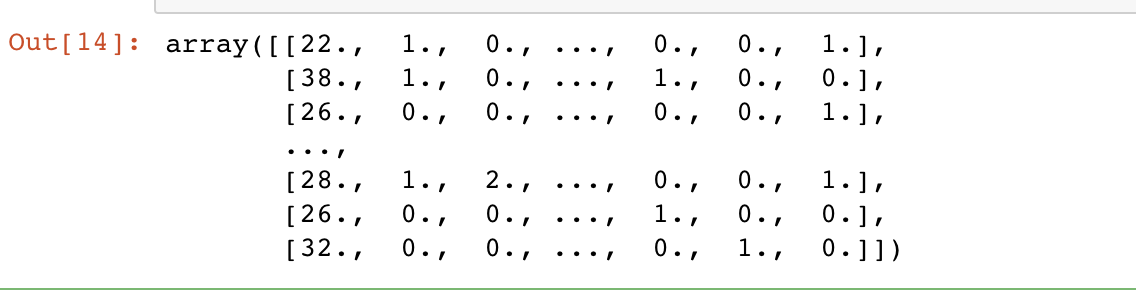``````y_train = train_set['Survived'] == 1
``````

### 2.构建模型

``````from sklearn.ensemble import RandomForestClassifier

forest_clf = RandomForestClassifier(random_state=42)

forest_clf.fit(X_train,y_train)
``````

## 五、模型评估

``````forest_clf.score(X_train,y_train)
``````## 六、方案实施

``````# 对测试数据集进行相同的数据预处理管道操作
X_test = preprocess_pipeline.fit_transform(test_set)
# 使用模型对数据进行预测
y_test = forest_clf.predict(X_test)

# 乘客id
passenger_id = test_set['PassengerId']
# 预测结果转换为int类型
y_test = y_test.astype(int)

# 将结果做为新的数据保存
pred_df = pd.DataFrame(
{ 'PassengerId': passenger_id ,
'Survived': y_test } )
pred_df.shape

# 保存到本地
pred_df.to_csv("./datasets/titanic/prediction.csv", index=False)
``````

#### 参考文章地址

• https://zhuanlan.zhihu.com/p/33565306
• https://zhuanlan.zhihu.com/p/27655949
• http://www.cnblogs.com/jasonfreak/p/5448385.html